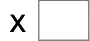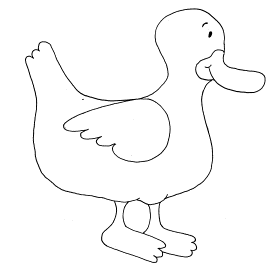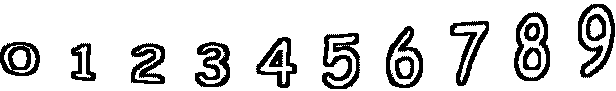7= 42   4= 326= 24   7= 63   6= 36   3= 3 6= 60   2= 10   2= 10   2= 6